# swarmchart3

3-D swarm scatter chart

Since R2020b

•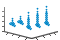## Syntax

``swarmchart3(x,y,z)``
``swarmchart3(x,y,z,sz)``
``swarmchart3(x,y,z,sz,c)``
``swarmchart3(___,mkr)``
``swarmchart3(___,'filled')``
``swarmchart3(tbl,xvar,yvar,zvar)``
``swarmchart3(tbl,xvar,yvar,zvar,'filled')``
``swarmchart3(ax,___)``
``swarmchart3(___,Name,Value)``
``s = swarmchart3(___)``

## Description

### Vector Data

example

````swarmchart3(x,y,z)` displays a 3-D swarm chart, which is a scatter plot with the points offset (jittered) in the `x`- and `y`-dimensions. The points form distinct shapes, and the outline of each shape is similar to a violin plot. 3-D swarm charts help you to visualize discrete (`x`,`y`) data with the distribution of the z data. At each (`x`,`y`) location, the points are jittered based on the kernel density estimate of `z`.```

example

````swarmchart3(x,y,z,sz)` specifies the marker sizes. To plot all the markers with the same size, specify `sz` as a scalar. To plot the markers with different sizes, specify `sz` as a vector that is the same size as `x`, `y`, and `z`.```

example

````swarmchart3(x,y,z,sz,c)` specifies the marker colors. To plot all the markers with the same color, specify `c` as a color name or an RGB triplet. To assign a different color to each marker, specify a vector the same size as `x`, `y`, and `z`. Alternatively, you can specify a three-column matrix of RGB triplets. The number of rows in the matrix must match the length of `x`, `y`, and `z`.```

example

````swarmchart3(___,mkr)` specifies a different marker than the default marker, which is a circle. Specify `mkr` after all the arguments in any of the previous syntaxes.```

example

````swarmchart3(___,'filled')` fills in the markers. Specify the `'filled'` option after all the arguments in any of the previous syntaxes.```

### Table Data

example

````swarmchart3(tbl,xvar,yvar,zvar)` plots the variables `xvar`, `yvar`, and `zvar` from the table `tbl`. To plot one data set, specify one variable each for `xvar`, `yvar`, and `zvar`. To plot multiple data sets, specify multiple variables for at least one of those arguments. The arguments that specify multiple variables must specify the same number of variables.```

example

````swarmchart3(tbl,xvar,yvar,zvar,'filled')` plots the specified variables from the table with filled circles.```

example

````swarmchart3(ax,___)` displays the swarm chart in the target axes. Specify the axes before all the arguments in any of the previous syntaxes.```
````swarmchart3(___,Name,Value)` specifies additional properties for the swarm chart using one or more `Name,Value` arguments. For example: `swarmchart3(x,y,z,'LineWidth',2)` creates a swarm chart with 2-point marker outlines.`swarmchart3(tbl,'MyX','MyY','MyZ','ColorVariable','MyColors')` creates a swarm chart from data in a table, and customizes the marker colors using data from the table. For a list of properties, see Scatter Properties.```

example

````s = swarmchart3(___)` returns the `Scatter` object. Use `s` to modify properties of the chart after creating it. For a list of properties, see Scatter Properties.```

## Examples

collapse all

Read the `BicycleCounts.csv` data set into a timetable called `tbl`. This data set contains bicycle traffic data over a period of time. Display the first five rows of `tbl`.

```tbl = readtable("BicycleCounts.csv"); tbl(1:5,:)```
```ans=5×5 table Timestamp Day Total Westbound Eastbound ___________________ _____________ _____ _________ _________ 2015-06-24 00:00:00 {'Wednesday'} 13 9 4 2015-06-24 01:00:00 {'Wednesday'} 3 3 0 2015-06-24 02:00:00 {'Wednesday'} 1 1 0 2015-06-24 03:00:00 {'Wednesday'} 1 1 0 2015-06-24 04:00:00 {'Wednesday'} 1 1 0 ```

Create a vector `x` with the day name from each observation.

```daynames = ["Sunday" "Monday" "Tuesday" "Wednesday" "Thursday" "Friday" "Saturday"]; x = categorical(tbl.Day,daynames);```

Create a categorical vector `y` containing the values `"pm"` or `"am"` according to the time for each observation in the table. Create vector `z` of eastbound traffic data. Then create a swarm chart of `x`, `y`, and `z`. The chart shows the data distributions for each morning and evening of the week.

```ispm = tbl.Timestamp.Hour < 12; y = categorical; y(ispm) = "pm"; y(~ispm) = "am"; z= tbl.Eastbound; swarmchart3(x,y,z);```Create vector `x` as a combination of zeros and ones, and create `y` as a vector containing all ones. Create `z` as a vector of squared random numbers. Then create a swarm chart of `x`, `y`, and `z`, and specify the size marker size as `5`.

```x = [zeros(1,500) ones(1,500)]; y = ones(1,1000); z = randn(1,1000).^2; swarmchart3(x,y,z,5);```Create vector `x` as a combination of zeros and ones, and create `y` as a vector containing all ones. Create `z` as a vector of squared random numbers. Then create a swarm chart of `x`, `y`, and `z`, and specify the point (`'.'`) marker symbol.

```x = [zeros(1,500) ones(1,500)]; y = ones(1,1000); z = randn(1,1000).^2; swarmchart3(x,y,z,'.');```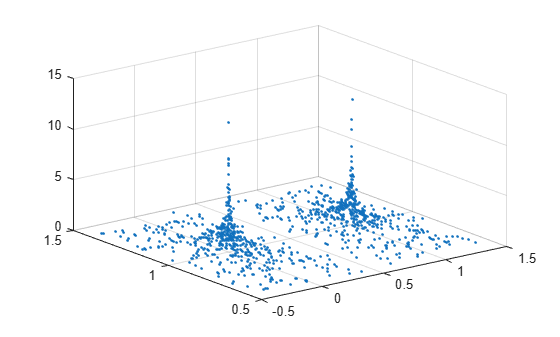Create vector `x` containing a combination of zeros and ones, and create `y` containing a random combination of ones and twos. Create `z` as a vector of squared random numbers. Specify the colors for the markers by creating vector `c` as the square root of `z`. Then create a swarm chart of `x`, `y`, and `z`. Set the marker size to `50` and specify the colors as `c`. The values in `c` index into the figure's colormap. Use the `'filled'` option to fill the markers with color instead of displaying them as hollow circles.

```x = [zeros(1,500) ones(1,500)]; y = randi(2,1,1000); z = randn(1,1000).^2; c = sqrt(z); swarmchart3(x,y,z,50,c,'filled');```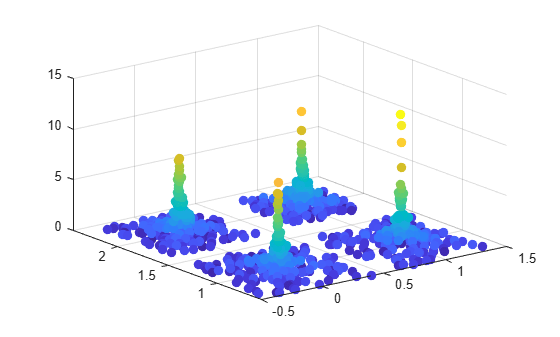Create vector `x` containing a combination of zeros and ones, and create `y` containing a random combination of the numbers one through four. Create `z` as a vector of squared random numbers. Then create a swarm chart of `x`, `y`, and `z` by calling the `swarmchart` function with a return argument that stores the `Scatter` object. Add x- and y-axis labels so you can see the effect of changing the jitter properties in each dimension.

```x = [zeros(1,500) ones(1,500)]; y = randi(4,1,1000); z = randn(1,1000).^2; s = swarmchart3(x,y,z); xlabel('X') ylabel('Y')```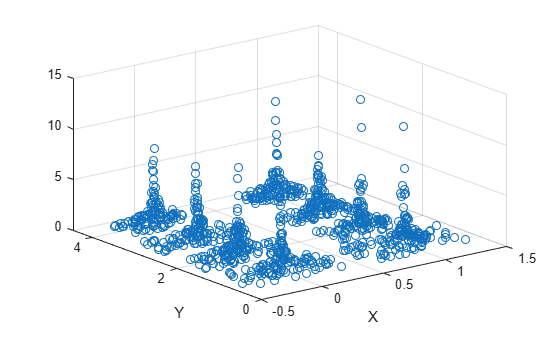Change the shapes of the clusters of points by setting the jitter properties on the `Scatter` object. In the `x` dimension, specify uniform random jitter, and change the jitter width to `0.5` data units. In the `y` dimension, specify normal random jitter, and change the jitter width to `0.1` data units. The spacing between points does not exceed the jitter width you specify.

```s.XJitter = 'rand'; s.XJitterWidth = 0.5; s.YJitter = 'randn'; s.YJitterWidth = 0.1;```A convenient way to plot data from a table is to pass the table to the `swarm3` function and specify the variables you want to plot. For example, create a table with four variables of random numbers, and plot the `X`, `Y1`, and `Z` variables. By default, the axis labels match the variable names.

```tbl = table(randi(2,100,1),randi(2,100,1),randi([10 11],100,1), ... randn(100,1),'VariableNames',{'X','Y1','Y2','Z'}); swarmchart3(tbl,'X','Y1','Z')```You can also plot multiple variables at the same time. For example, plot `Y1` and `Y2` on the y-axis by specifying the `yvar` argument as the cell array `{'Y1','Y2'}`. Then add a legend. The legend labels match the variable names.

```swarmchart3(tbl,'X',{'Y1','Y2'},'Z') legend```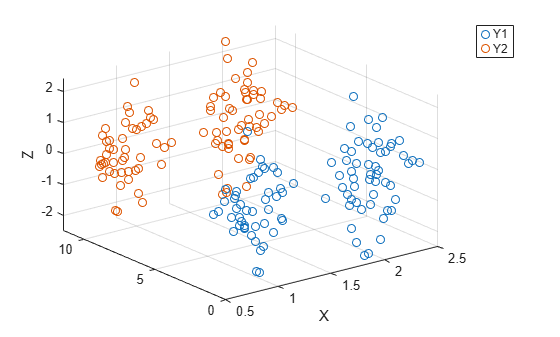One way to plot data from a table and customize the colors and marker sizes is to set the `ColorVariable` and `SizeData` properties. You can set these properties as name-value arguments when you call the `swarmchart3` function, or you can set them on the `Scatter` object later.

For example, create a table with four variables of random numbers, and plot the `X`, `Y`, and `Z` variables with filled markers. Vary the marker colors by specifying the `ColorVariable` name-value argument. Return the `Scatter` object as `s`, so you can set other properties later.

```tbl = table(randi(2,100,1),randn(100,1),randn(100,1),randn(100,1), ... 'VariableNames',{'X','Y','Z','Colors'}); s = swarmchart3(tbl,'X','Y','Z','filled','ColorVariable','Colors');```Change the marker sizes to 100 points by setting the `SizeData` property.

`s.SizeData = 100;`Read the `BicycleCounts.csv` data set into a timetable called `tbl`. This data set contains bicycle traffic data over a period of time. Display the first five rows of `tbl`.

```tbl = readtable("BicycleCounts.csv"); tbl(1:5,:)```
```ans=5×5 table Timestamp Day Total Westbound Eastbound ___________________ _____________ _____ _________ _________ 2015-06-24 00:00:00 {'Wednesday'} 13 9 4 2015-06-24 01:00:00 {'Wednesday'} 3 3 0 2015-06-24 02:00:00 {'Wednesday'} 1 1 0 2015-06-24 03:00:00 {'Wednesday'} 1 1 0 2015-06-24 04:00:00 {'Wednesday'} 1 1 0 ```

Create vector `x` with the days names for each observation. Create a categorical vector `y` containing the values `"pm"` or `"am"` according to the time for each observation in the table. Define `ze` as a vector of eastbound traffic data, and define `zw` as a vector of westbound traffic data.

```daynames = ["Sunday" "Monday" "Tuesday" "Wednesday" "Thursday" "Friday" "Saturday"]; x = categorical(tbl.Day,daynames); ispm = tbl.Timestamp.Hour<12; y = categorical; y(ispm) = 'pm'; y(~ispm) = 'am'; ze = tbl.Eastbound; zw = tbl.Westbound;```

Create a tiled chart layout in the `'flow'` tile arrangement, so that the axes fill the available space in the layout. Call the `nexttile` function to create an axes object and return it as `ax1`. Then create a swarm chart of the eastbound data by passing `ax1` to the `swarmchart` function.

```tiledlayout('flow') ax1=nexttile; swarmchart3(ax1,x,y,ze,'.');```Repeat the process to create a second axes object and a swarm chart for the westbound traffic.

```ax2 = nexttile; z = tbl.Westbound; swarmchart3(ax2,x,y,zw,'.');```## Input Arguments

collapse all

x-coordinates, specified as a numeric scalar or a vector the same size as `y` and `z`.

Data Types: `single` | `double` | `int8` | `int16` | `int32` | `int64` | `uint8` | `uint16` | `uint32` | `uint64` | `categorical`

y-coordinates, specified as a numeric scalar or a vector the same size as `x` and `z`.

Data Types: `single` | `double` | `int8` | `int16` | `int32` | `int64` | `uint8` | `uint16` | `uint32` | `uint64` | `categorical`

z-coordinates, specified as a numeric scalar or a vector the same size as `x` and `y`.

Data Types: `single` | `double` | `int8` | `int16` | `int32` | `int64` | `uint8` | `uint16` | `uint32` | `uint64` | `datetime` | `duration`

Marker size in points, specified in one of these forms:

• Numeric scalar — Plot all markers with equal size.

• Row or column vector — Use different sizes for each marker. The length of `sz` must equal the length of `x`, `y`, and `z`.

• `[]` — Use the default size of 36 points.

Marker color, specified in one of these forms:

• RGB triplet or color name — Plot all the markers with the same color. An RGB triplet is a three-element row vector whose elements specify the intensities of the red, green, and blue components of the color. The intensities must be in the range `[0,1]`. Alternatively, you can specify a color name from the table below.

• Three column matrix of RGB triplets — Use different colors for each marker. Each row of the matrix specifies an RGB triplet color for the corresponding marker. The number of rows must equal the length of `x`, `y`, and `z`.

• Vector — Use different colors for each marker. The values in `c` index into the current colormap, and they cover the full range of the colormap. The length of `c` must equal the length of `x`, `y`, and `z`. To change the colormap, use the `colormap` function.

Color NameDescriptionEquivalent RGB Triplet
`'red'` or `'r'`Red`[1 0 0]`
`'green'` or `'g'`Green`[0 1 0]`
`'blue'` or `'b'`Blue`[0 0 1]`
`'yellow'` or `'y'`Yellow`[1 1 0]`
`'magenta'` or `'m'`Magenta`[1 0 1]`
`'cyan'` or `'c'`Cyan`[0 1 1]`
`'white'` or `'w'`White`[1 1 1]`
`'black'` or `'k'`Black`[0 0 0]`

Marker type, specified as one of the values listed in this table.

MarkerDescriptionResulting Marker
`"o"`Circle`"+"`Plus sign`"*"`Asterisk`"."`Point`"x"`Cross`"_"`Horizontal line`"|"`Vertical line`"square"`Square`"diamond"`Diamond`"^"`Upward-pointing triangle`"v"`Downward-pointing triangle`">"`Right-pointing triangle`"<"`Left-pointing triangle`"pentagram"`Pentagram`"hexagram"`HexagramOption to fill the interior of the markers, specified as `'filled'`. Use this option with markers that have a face, for example, `'o'` or `'square'`. Markers that do not have a face and contain only edges do not render at all (`'+'`, `'*'`, `'.'`, and `'x'`).

The `'filled'` option sets the `MarkerFaceColor` property of the `Scatter` object to `'flat'` and the `MarkerEdgeColor` property to `'none'`. In this case, MATLAB® draws the marker faces, but not the edges.

Source table containing the data to plot, specified as a table or a timetable.

Table variables containing the x-coordinates, specified as one or more table variable indices.

#### Specifying Table Indices

Use any of the following indexing schemes to specify the desired variable or variables.

Indexing SchemeExamples

Variable names:

• `"A"` or `'A'` — A variable called `A`

• `["A","B"]` or `{'A','B'}` — Two variables called `A` and `B`

• `"Var"+digitsPattern(1)` — Variables named `"Var"` followed by a single digit

Variable index:

• An index number that refers to the location of a variable in the table.

• A vector of numbers.

• A logical vector. Typically, this vector is the same length as the number of variables, but you can omit trailing `0` or `false` values.

• `3` — The third variable from the table

• `[2 3]` — The second and third variables from the table

• `[false false true]` — The third variable

Variable type:

• `vartype("categorical")` — All the variables containing categorical values

The table variables you specify can contain numeric, categorical, datetime, or duration values.

To plot one data set, specify one variable for `xvar`, one variable for `yvar`, and one variable for `zvar`. For example, create a table with four variables of normally distributed random values. Plot the `X`, `Y1`, and `Z` variables.

```tbl = table(randn(100,1),randn(100,1),randn(100,1)+5,randn(100,1), ... 'VariableNames',{'X','Y1','Y2','Z'}); swarmchart3(tbl,'X','Y1','Z')```

To plot multiple data sets together, specify multiple variables for at least one of `xvar`, `yvar`, or `zvar`. If you specify multiple variables for more than one argument, the number of variables must be the same for each of those arguments.

For example, plot the `X` variable on the x-axis, the `Y1` and `Y2` variables on the y-axis, and the `Z` variable on the z-axis.

`swarmchart3(tbl,'X',{'Y1','Y2'},'Z')`

You can also use different indexing schemes for `xvar`, `yvar`, and `zvar`. For example, specify `xvar` as a variable name, `yvar` as an index number, and `zvar` as a logical vector.

`swarmchart3(tbl,'X',2,[false false true])`

Table variables containing the y-coordinates, specified as one or more table variable indices.

#### Specifying Table Indices

Use any of the following indexing schemes to specify the desired variable or variables.

Indexing SchemeExamples

Variable names:

• `"A"` or `'A'` — A variable called `A`

• `["A","B"]` or `{'A','B'}` — Two variables called `A` and `B`

• `"Var"+digitsPattern(1)` — Variables named `"Var"` followed by a single digit

Variable index:

• An index number that refers to the location of a variable in the table.

• A vector of numbers.

• A logical vector. Typically, this vector is the same length as the number of variables, but you can omit trailing `0` or `false` values.

• `3` — The third variable from the table

• `[2 3]` — The second and third variables from the table

• `[false false true]` — The third variable

Variable type:

• `vartype("categorical")` — All the variables containing categorical values

The table variables you specify can contain numeric, categorical, datetime, or duration values.

To plot one data set, specify one variable for `xvar`, one variable for `yvar`, and one variable for `zvar`. For example, create a table with four variables of normally distributed random values. Plot the `X`, `Y1`, and `Z` variables.

```tbl = table(randn(100,1),randn(100,1),randn(100,1)+5,randn(100,1), ... 'VariableNames',{'X','Y1','Y2','Z'}); swarmchart3(tbl,'X','Y1','Z')```

To plot multiple data sets together, specify multiple variables for at least one of `xvar`, `yvar`, or `zvar`. If you specify multiple variables for more than one argument, the number of variables must be the same for each of those arguments.

For example, plot the `X` variable on the x-axis, the `Y1` and `Y2` variables on the y-axis, and the `Z` variable on the z-axis.

`swarmchart3(tbl,'X',{'Y1','Y2'},'Z')`

You can also use different indexing schemes for `xvar`, `yvar`, and `zvar`. For example, specify `xvar` as a variable name, `yvar` as an index number, and `zvar` as a logical vector.

`swarmchart3(tbl,'X',2,[false false true])`

Table variables containing the z-coordinates, specified as one or more table variable indices.

#### Specifying Table Indices

Use any of the following indexing schemes to specify the desired variable or variables.

Indexing SchemeExamples

Variable names:

• `"A"` or `'A'` — A variable called `A`

• `["A","B"]` or `{'A','B'}` — Two variables called `A` and `B`

• `"Var"+digitsPattern(1)` — Variables named `"Var"` followed by a single digit

Variable index:

• An index number that refers to the location of a variable in the table.

• A vector of numbers.

• A logical vector. Typically, this vector is the same length as the number of variables, but you can omit trailing `0` or `false` values.

• `3` — The third variable from the table

• `[2 3]` — The second and third variables from the table

• `[false false true]` — The third variable

Variable type:

• `vartype("categorical")` — All the variables containing categorical values

The table variables you specify can contain numeric, categorical, datetime, or duration values.

To plot one data set, specify one variable for `xvar`, one variable for `yvar`, and one variable for `zvar`. For example, create a table with four variables of normally distributed random values. Plot the `X`, `Y1`, and `Z` variables.

```tbl = table(randn(100,1),randn(100,1),randn(100,1)+5,randn(100,1), ... 'VariableNames',{'X','Y1','Y2','Z'}); swarmchart3(tbl,'X','Y1','Z')```

To plot multiple data sets together, specify multiple variables for at least one of `xvar`, `yvar`, or `zvar`. If you specify multiple variables for more than one argument, the number of variables must be the same for each of those arguments.

For example, plot the `X` variable on the x-axis, the `Y1` and `Y2` variables on the y-axis, and the `Z` variable on the z-axis.

`swarmchart3(tbl,'X',{'Y1','Y2'},'Z')`

You can also use different indexing schemes for `xvar`, `yvar`, and `zvar`. For example, specify `xvar` as a variable name, `yvar` as an index number, and `zvar` as a logical vector.

`swarmchart3(tbl,'X',2,[false false true])`

Target axes, specified as an `Axes` object. If you do not specify the axes, MATLAB plots into the current axes, or it creates an `Axes` object if one does not exist.

### Name-Value Arguments

Specify optional pairs of arguments as `Name1=Value1,...,NameN=ValueN`, where `Name` is the argument name and `Value` is the corresponding value. Name-value arguments must appear after other arguments, but the order of the pairs does not matter.

Before R2021a, use commas to separate each name and value, and enclose `Name` in quotes.

Example: `swarmchart3(randi(2,500,1),randi(2,500,1),randn(500,1),'MarkerFaceColor','red')` specifies red filled markers.

Type of jitter (spacing of points) along the x-dimension, specified as one of the following values:

• `'none'` — Do not jitter the points.

• `'density'` — Jitter the points using the kernel density estimate of y for 2-D charts. If you specify this option in two dimensions for a 3-D chart, the points are jittered based on the kernel density estimate in the third dimension. For example, setting `XJitter` and `YJitter` to `'density'` uses the kernel density estimate of z.

• `'rand'` — Jitter the points randomly with a uniform distribution.

• `'randn'` — Jitter points randomly with a normal distribution.

Maximum amount of jitter (offset between points) along the x-dimension, specified as a nonnegative scalar value in data units.

For example, to set the jitter width to 90% of the shortest distance between adjacent points, take the minimum distance between unique values of `x` and scale by `0.9`.

`XJitterWidth = 0.9 * min(diff(unique(x)));`

Type of jitter (spacing of points) along the y-dimension, specified as one of the following values:

• `'none'` — Do not jitter the points.

• `'density'` — Jitter the points using the kernel density estimate of x for 2-D charts. If you specify this option in two dimensions for a 3-D chart, the points are jittered based on the kernel density estimate in the third dimension. For example, setting `XJitter` and `YJitter` to `'density'` uses the kernel density estimate of z.

• `'rand'` — Jitter the points randomly with a uniform distribution.

• `'randn'` — Jitter points randomly with a normal distribution.

Maximum amount of jitter (offset between points) along the y-dimension, specified as a nonnegative scalar value in data units.

For example, to set the jitter width to 90% of the shortest distance between adjacent points, take the minimum distance between unique values of `y` and scale by `0.9`.

`YJitterWidth = 0.9 * min(diff(unique(y)));`

Table variable containing the color data, specified as a variable index into the source table.

#### Specifying the Table Index

Use any of the following indexing schemes to specify the desired variable.

Indexing SchemeExamples

Variable name:

• A string scalar or character vector.

• A `pattern` object. The pattern object must refer to only one variable.

• `"A"` or `'A'` — A variable called `A`

• `"Var"+digitsPattern(1)` — The variable with the name `"Var"` followed by a single digit

Variable index:

• An index number that refers to the location of a variable in the table.

• A logical vector. Typically, this vector is the same length as the number of variables, but you can omit trailing `0` or `false` values.

• `3` — The third variable from the table

• `[false false true]` — The third variable

Variable type:

• A `vartype` subscript that selects a table variable of a specified type. The subscript must refer to only one variable.

• `vartype("double")` — The variable containing double values

#### Specifying Color Data

Specifying the `ColorVariable` property controls the colors of the markers. The data in the variable controls the marker fill color when the `MarkerFaceColor` property is set to `"flat"`. The data can also control the marker outline color, when the `MarkerEdgeColor` is set to `"flat"`.

The table variable you specify can contain values of any numeric type. The values can be in either of the following forms:

• A column of numbers that linearly map into the current colormap.

• A three-column array of RGB triplets. RGB triplets are three-element vectors whose values specify the intensities of the red, green, and blue components of specific colors. The intensities must be in the range `[0,1]`. For example, `[0.5 0.7 1]` specifies a shade of light blue.

When you set the `ColorVariable` property, MATLAB updates the `CData` property.

## Algorithms

The points in a swarm chart are jittered using uniform random values that are weighted by the Gaussian kernel density estimate of `z` and the relative number of points at each (`x`, `y`) location. This behavior corresponds to the default `'density'` setting of the `XJitter` and `YJitter` properties on the `Scatter` object when you call the `swarmchart3` function.

The maximum spread of points at each `x` location is 90% of the smallest distance between adjacent points by default. For example, in the `x` dimension, the spread is calculated as:

`spread = 0.9 * min(diff(unique(x)));`

You can control the offset by setting the `XJitterWidth` and `YJitterWidth` properties on the `Scatter` object.

## Version History

Introduced in R2020b

expand all